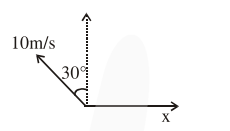# A person is swimming with a speed of`
Question:

A person is swimming with a speed of $10 \mathrm{~m} /$ s at an angle of $120^{\circ}$ with the flow and reaches to a point directly opposite on the other side of the river. The speed of the flow is ' $x$ ' $\mathrm{m} / \mathrm{s}$. The value of ' $x$ ' to the nearest integer is___________.

Solution:$10 \sin 30^{\circ}=x$

$x=5 \mathrm{~m} / \mathrm{s}$